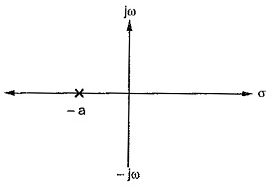### Circuit & Field Theory - short answer questions from AMIE exams (Winter 2018)

Answer the following in the brief.

Define half-power frequencies in resonant RLC series circuit
If the series RLC circuit is driven by a variable frequency at a constant voltage, then the magnitude of the current, I is proportional to the impedance, Z, therefore at resonance the power absorbed by the circuit must be at its maximum value as P = I²Z.
If we now reduce or increase the frequency until the average power absorbed by the resistor in the series resonance circuit is half that of its maximum value at resonance, we produce two frequency points called the half-power points which are -3dB down from maximum, taking 0dB as the maximum current reference.
The point corresponding to the lower frequency at half the power is called the “lower cut-off frequency”, labelled fL with the point corresponding to the upper frequency at half power being called the “upper cut-off frequency”, labelled fH. The distance between these two points, i.e. ( fH – fL ) is called the Bandwidth, (BW) and is the range of frequencies over which at least half of the maximum power and current is provided as shown.

What is the constant flux linkage theorem? Give its application.
The constant flux linkage theorem states that flux linkages in an inductor cannot change abruptly.
The theorem depends on the well-known fact that the magnetic flux linked with any closed circuit cannot change instantaneously with a sudden change of applied voltage so that the sum of the magnetic flux linkages will remain constant if the voltage subsequent to change and the circuit losses are zero.

Explain how the time-domain behaviour of a circuit may be obtained from its pole-zero plot.
From the locations of poles and zeros of the network function in the s-plane, the time response of the network can be perfectly identified.Pole locationExponential location

Define pole and zero frequencies of a network function.
• Poles and Zeros of a transfer function are the frequencies for which the value of the denominator and numerator of transfer function becomes zero respectively. The values of the poles and the zeros of a system determine whether the system is stable, and how well the system performs. Control systems, in the most simple sense, can be designed simply by assigning specific values to the poles and zeros of the system.
• The poles capture the ‘form’ of each 'component’ of a signal’s ‘structure’ or a system’s response in the time domain.
• The role of the zeros is somewhat more subtle but very important nonetheless. They determine the way in which these components are ‘mixed’ in the time domain, i.e. they determine the phase and magnitude of each component ‘generated’ by each pole.

Explain the concept of complex frequency.
A type of frequency that depends on two parameters; one is the σ which controls the
magnitude of the signal and the other is “w”, which controls the rotation of the signal; is known as “complex frequency”.
s = σ + jw

"s" is a generalized frequency variable whose real part a describes growth and decay of the amplitudes of signals, and whose imaginary part jω is the angular frequency in the usual sense.

Define Gauss’s law in electrostatic.
Gauss’s law for electricity states that the electric flux across any closed surface is proportional to the net electric charge enclosed by the surface. The law implies that isolated electric charges exist and that like charges repel one another while unlike charges attract.

State uniqueness theorem.
The uniqueness theorem states that if we can find a solution that satisfies Laplace's equation and the boundary condition

this is the only solution.

When a magnetic field is an incident on a coil of conductor, the magnitude of the electromotive force (EMF) induced in the coil is directly proportional to the rate of change in the inducing magnetic field and dot product between the field direction and the axis of the coil.
Lenz’s law
When a magnetic field induces a current in a conducting coil, the induced current also generates its own magnetic field that points opposite to the inducing magnetic field.

Define divergence and curl of a vector.
See Curl of vector at this link
The Divergence of a vector field is a measure of the net flow of the flux around a given point. The divergence of vector field at a given point is the net outward flux per unit volume as the volume shrinks (tends to) zero at that point.
The simplest synonym for the divergence is "outgoingness". More is the divergence more are the field lines coming outside around that point and it should be considered as positive divergence. In contrast to that, if field lines tend to come inside then we can say the field is accumulating at that point which is opposite to "outgoingness" and hence considered as a negative divergence.
Divergence can be written as

Express Z parameters of a two-port network in terms of Y parameters.

---
The study material for AMIE/B Tech/Junior Engineer exams is available at https://amiestudycircle.com